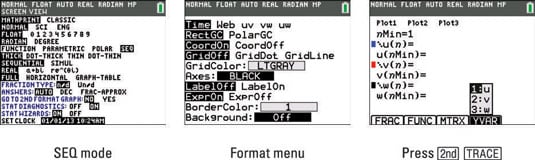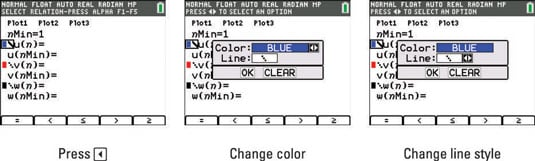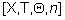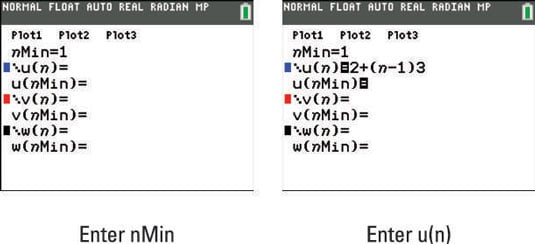##### TI-84 Plus CE Graphing Calculator For Dummies, 3rd EditionSequences make interesting graphs! You need to learn how to set the mode and select the color before entering a sequence in your TI-84 Plus calculator. Then, you can have the fun of graphing a sequence.

## Changing the mode

You can’t begin graphing sequences until you change the mode of your calculator. Follow these steps to change the mode of your calculator:

1. Press [MODE] and put the calculator in SEQ mode.

To highlight an item in the Mode menu, use the arrow keys to place the cursor on the item, and then press [ENTER]. Highlight SEQ in the fifth line to put the calculator in Sequence mode. See the first screen.The top line in the Format menu is where you choose the type of sequence plot you want to graph. Here are the five choices:

• Time: Time plots are discreet graphs (meaning the points are unconnected) of sequences. This is the most popular type of plot. The independent variable, n, will be on the x-axis and u(n), v(n), or w(n) will be on the y-axis.

• Web: Web plots are used to study recursive sequences that converge, diverge, or oscillate. A web plot enables you to see the behavior of the sequence over the long-term. The x-axis is one of the recursive variables, u(n–1), v(n–1), or w(n–1). The y-axis is u(n), v(n), or w(n).

• uv, vw, and uw: Phase plots are used to show relationships between two sequences. uv has an x-axis of u(n) and y-axis v(n). vw has an x-axis of v(n) and y-axis w(n). uw has an x-axis of u(n) and y-axis w(n).

On a TI-84 Plus C, press [ALPHA][TRACE] to enter the variables u, v, and w as shown in the third screen. If you are using a TI-84 Plus, press [2nd] followed by the , , or  key.

## Selecting the color

All the functionality that you are used to having in Function mode is also available in Sequence mode. If you would like to customize your graph by changing the color or the line style, follow these steps:

1. Press [Y=] to access the Y= editor.

2. Press the left-arrow key to navigate your cursor to the left of u(n).

See the first screen. Graphing a sequence on your calculator requires using up to three lines in the Y= editor.3. Press [ENTER] and use the left- and right-arrow keys to change the color using the spinner menu.

See the second screen.

4. Press [ENTER] and use the left- and right-arrow keys to change the line style using the spinner menu.

Keep the line style at Dotted–Thick or change to Dotted–Thin. Time plots should be discreet graphs.

5. Press [ENTER] twice to make the changes effective.

## Entering sequences

If you are an observant person, then you may have noticed the Y= editor looks quite different than you may be used to. It seems like Y1 has been replaced with three equations, nMin, u(n), and u(nMin). Remember, the x and y variables are now defined in terms of a new parameter, n. When you pressin Sequence mode, an n appears instead of an x.

Here are a few terms that you need to begin sequence graphing:

• n: The independent variable.

• nMin: Where n starts counting (usually at 1).

• u(n): This is the function that generates the sequence.

• u(nMin): The value of the initial term. This is not necessary to include unless you are dealing with recursive sequences. If you need to include more than one term, use {} and enter the terms as a list.

• u(n–1): Previous term, used in recursive sequences.

• u(n–2): Term before the previous term, used in recursive sequences.

For this exercise, the following arithmetic sequence was used: 2, 5, 8, . . .

Explicit formula for an arithmetic sequence: an = a1+(n–1)d

The explicit formula for this sequence is an = 2+(n–1)3. Once you have found the explicit formula for a particular sequence, you can enter the sequence in your calculator by following these steps:

1. Press [Y=] to access the Y= editor.

2. Enter a value for nMin.

nMin is the value where n starts counting. See the first screen.3. Press [ENTER] or press the down-arrow key to navigate to the next line.

4. Enter the explicit formula for u(n).

See the second screen. Don’t forget to pressfor n.

5. Press [ENTER] or press the down-arrow key to navigate to the next line.

6. Enter u(nMin) if necessary.

Only enter a value for the initial term if it is a recursive sequence.